Martin McBride, 2020-08-14
Tags quadrilateral square rectangle parallelogram rhombus trapezium isosceles trapezium kite
Categories maths gcse geometry

A quadrilateral is a shape with four straight sides. There are various types of quadrilateral.

## Squares

An square is a is a quadrilateral where all four sides are equal, and all four angles are 90 degrees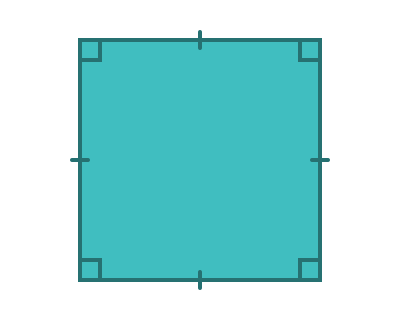The tick marks on the four sides indicate that the sides are the same length.

The square angle symbols in the corners indicate that each corner is a 90 degree angle.

## Rectangles

A rectangle is a quadrilateral that has all four angles equal to 90 degrees.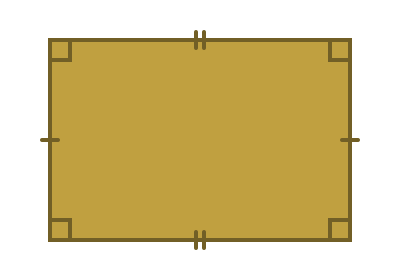The opposite sides of a rectangle are always equal.

A square is a special type of rectangle, where all four sides are equal.

## Parallelograms

A parallelogram is a quadrilateral where both pairs of opposite sides are parallel. You can think of a parallelogram as a "pushed over" rectangle.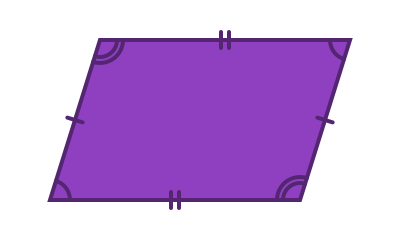The opposite sides of a parallelogram have equal length.

The diagonally opposite angles are equal.

A rectangle is a special type of parallelogram where all 4 angles are 90 degrees.

## Rhombus

A rhombus is a quadrilateral where all four sides are the same length, and both pairs of opposite sides are parallel. You can think of a rhombus as a "pushed over" square.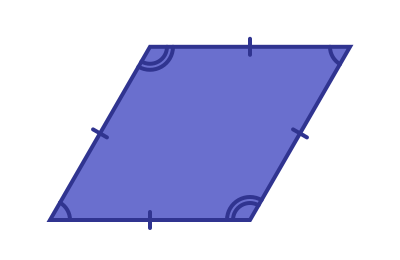A rhombus is a special type of parallelogram where all four sides are equal. The diagonally opposite angles of a rhombus are equal.

If you rotate a rhombus you will see a shape that is sometimes called a diamond. It is the same shape, just rotated.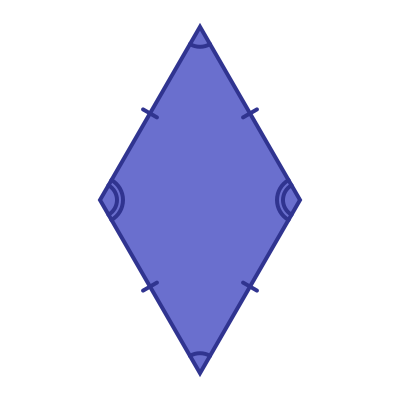## Trapezium

A trapezium is a quadrilateral that has at least two parallel sides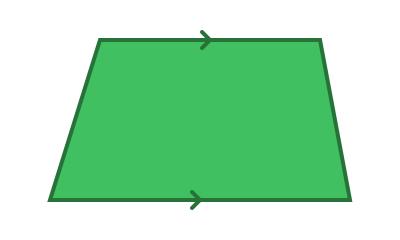An isosceles trapezium is one where the two sides make the same angle to the base (but with opposite direction of slope)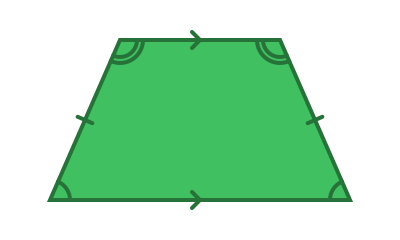In that case, the two sides are of equal length.

## Kite

A kite is a quadrilateral that has two pairs of equal length sides that are adjacent to each other (that is, next to each other).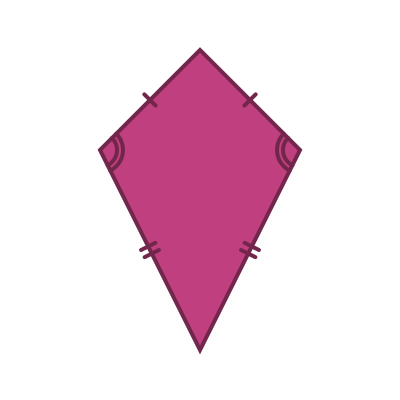The opposite angles of a kite are equal, as shown.

A rhombus is a special case of a kite where the lengths of all four sides are equal.